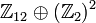# Group cohomology of symmetric groups

View group cohomology of group families | View other specific information about symmetric group

## Particular cases$n$$n!$ (order of symmetric group) Symmetric group of degree$n$ Group cohomology page
1 1 trivial group group cohomology of trivial group
2 2 cyclic group:Z2 group cohomology of cyclic group:Z2
3 6 symmetric group:S3 group cohomology of symmetric group:S3
4 24 symmetric group:S4 group cohomology of symmetric group:S4
5 120 symmetric group:S5 group cohomology of symmetric group:S5
6 720 symmetric group:S6 group cohomology of symmetric group:S6

## Classifying space and corresponding chain complex

The homology and cohomology groups of the symmetric group$S_n$ are the same as the respective homology and cohomology groups of the configuration space of$n$ unordered points in a countable-dimensional real projective space. For more on the topological perspective, see configuration space of unordered points of a countable-dimensional real projective space on the Topospaces wiki.

## Homology groups for trivial group action

FACTS TO CHECK AGAINST (homology group for trivial group action):
First homology group: first homology group for trivial group action equals tensor product with abelianization
Second homology group: formula for second homology group for trivial group action in terms of Schur multiplier and abelianization|Hopf's formula for Schur multiplier
General: universal coefficients theorem for group homology|homology group for trivial group action commutes with direct product in second coordinate|Kunneth formula for group homology

### Over the integers

Here, "0" for a group is shorthand for the trivial group.$\mathbb{Z}_m$ is shorthand for the finite cyclic group$\mathbb{Z}/m\mathbb{Z}$.

The homology groups eventually stabilize in the following sense: for any fixed$q$, there exists a large enough$n$ (explicit expression for$n$ in terms of$q$ -- around double?) such that$H_q(S_m;\mathbb{Z}) \cong H_q(S_n;\mathbb{Z})$ for all$m \ge n$. The corresponding stable value of homology group is termed the stable homology group of degree$q$ for the symmetric groups.$n$$n!$ symmetric group$S_n$ of degree$n$ if a finite group with periodic cohomology, period of sequence of homology groups for positive degrees$H_1(S_n;\mathbb{Z})$$H_2(S_n;\mathbb{Z})$$H_3(S_n;\mathbb{Z})$$H_4(S_n;\mathbb{Z})$$H_5(S_n;\mathbb{Z})$$H_6(S_n;\mathbb{Z})$$H_7(S_n;\mathbb{Z})$$H_8(S_n;\mathbb{Z})$$H_9(S_n;\mathbb{Z})$$H_{10}(S_n;\mathbb{Z})$
0 1 trivial group 1 0 0 0 0 0 0 0 0 0 0
1 1 trivial group 1 0 0 0 0 0 0 0 0 0 0
2 2 cyclic group:Z2 2$\mathbb{Z}_2$ 0$\mathbb{Z}_2$ 0$\mathbb{Z}_2$ 0$\mathbb{Z}_2$ 0$\mathbb{Z}_2$ 0
3 6 symmetric group:S3 4$\mathbb{Z}_2$ 0$\mathbb{Z}_6$ 0$\mathbb{Z}_2$ 0$\mathbb{Z}_6$ 0$\mathbb{Z}_2$ 0
4 24 symmetric group:S4 --$\mathbb{Z}_2$$\mathbb{Z}_2$$\mathbb{Z}_{12} \oplus \mathbb{Z}_2$$\mathbb{Z}_2$$(\mathbb{Z}_2)^3$$(\mathbb{Z}_2)^2$$\mathbb{Z}_{12} \oplus (\mathbb{Z}_2)^2$$(\mathbb{Z}_2)^3$$(\mathbb{Z}_2)^4$$(\mathbb{Z}_2)^3$
5 120 symmetric group:S5 --$\mathbb{Z}_2$$\mathbb{Z}_2$$\mathbb{Z}_{12} \oplus \mathbb{Z}_2$$\mathbb{Z}_2$$(\mathbb{Z}_2)^3$$(\mathbb{Z}_2)^2$$\mathbb{Z}_{60} \oplus (\mathbb{Z}_2)^2$$(\mathbb{Z}_2)^3$$(\mathbb{Z}_2)^4$$(\mathbb{Z}_2)^3$
6 720 symmetric group:S6 --$\mathbb{Z}_2$$\mathbb{Z}_2$$\mathbb{Z}_{12} \oplus \mathbb{Z}_2 \oplus \mathbb{Z}_2$$\mathbb{Z}_2 \oplus \mathbb{Z}_2$$(\mathbb{Z}_2)^5$$(\mathbb{Z}_2)^5$$\mathbb{Z}_{60} \oplus \mathbb{Z}_6 \oplus (\mathbb{Z}_2)^5$$(\mathbb{Z}_2)^8$
7 5040 symmetric group:S7 --$\mathbb{Z}_2$$\mathbb{Z}_2$$\mathbb{Z}_{12} \oplus \mathbb{Z}_2 \oplus \mathbb{Z}_2$$\mathbb{Z}_2 \oplus \mathbb{Z}_2$$(\mathbb{Z}_2)^5$$(\mathbb{Z}_2)^5$
Stable -- -- --$\mathbb{Z}_2$$\mathbb{Z}_2$$\mathbb{Z}_{12} \oplus \mathbb{Z}_2 \oplus \mathbb{Z}_2$  ?  ?  ?  ?  ?  ?  ?